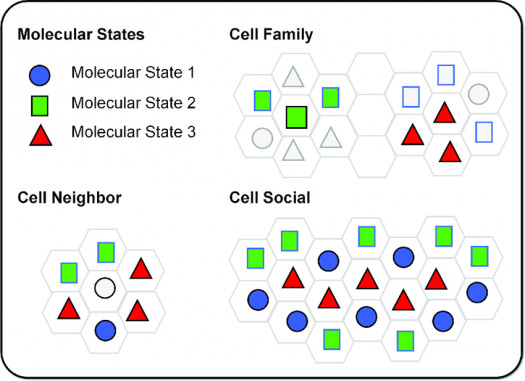# What Do You Know About Molecular State

10 QuestionsSettingsIn physics and chemistry, molecular term symbol is a short-form definition of the group representation and angular momenta that defines the state of a molecule. Take a quiz and learn more about molecular state

Related Topics
• 1.
Molecular State is the equivalent of the term symbol for the?
• A.

Atomic case

• B.

Molecules

• C.

Atomic State

• D.

None of the above

• 2.
Molecular State presentation is restricted to the case of homonuclear diatomic?
• A.

Mass

• B.

Molecules

• C.

Proton

• D.

Electron

• 3.
In the case of less symmetric molecules, the molecular term symbol contains the symbol of the?
• A.

Molecule

• B.

Group representation

• C.

Diatomic

• D.

None of the above

• 4.
In a molecular state form, S is the total spin?
• A.

Diatomic

• B.

Monolithic

• C.

Quantum number

• D.

None of the above

• 5.
The Omega symbol is the projection of total angular momentum along the internuclear?
• A.

Point

• B.

Region

• C.

Scale

• D.

Axis

• 6.
The plus or minus (+/-) is the reflection symmetry along an?
• A.

Arbitrary plane

• B.

Quantum place

• C.

Axis

• D.

None of the above

• 7.
The cylindrical symmetry of a linear molecule ensure positives and negative?
• A.

Values

• B.

Axis

• C.

Diatomic

• D.

Scale

• 8.
In a molecular state, the molecular orbital will be degenerate in the absence of spin-orbit?
• A.

Coupling

• B.

Axis

• C.

Region

• D.

Scale

• 9.
Spin-orbit coupling lifts the degeneracy of the electronic?
• A.

Direction

• B.

Motion

• C.

Diatomic

• D.

State

• 10.
In a molecular state, there is an infinite number of planes containing the?
• A.

Internuclear axis

• B.

Molecular axis

• C.

Perpendicular axis

• D.

None of the above